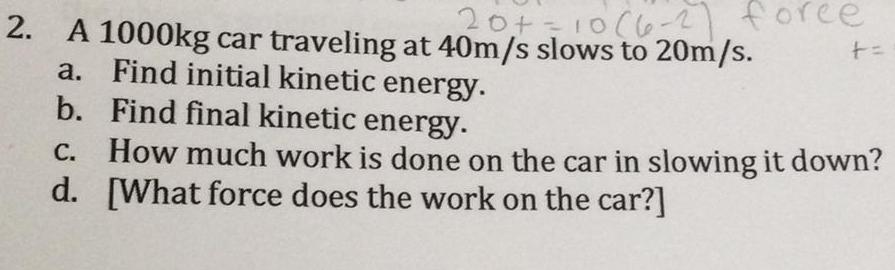Question:

# 2. A 1000kg car traveling at 40m/s slows to 20m/s. 20+ = 10 (6-27 force Find initial kinetic energy. a. b. + = Find final kinetic energy. c. How much work is done on the car in slowing it down? d. [What force does the work on the car?]

Last updated: 8/8/20222. A 1000kg car traveling at 40m/s slows to 20m/s. a. Find initial kinetic energy. b. Find final kinetic energy. c. How much work is done on the car in slowing it down? d. [What force does the work on the car?]